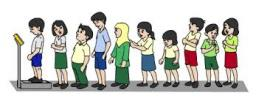# Fifty-six 5763

Fifty-six pupils attend the eighth grade of primary school. There are 18 more boys than girls. How many boys and girls are in the eighth grade of primary school?

c =  37
d =  19

### Step-by-step explanation:

c+d=56
c = 18+d

c+d = 56
c-d = 18

Row 2 - Row 1 → Row 2
c+d = 56
-2d = -38

d = -38/-2 = 19
c = 56-d = 56-19 = 37

c = 37
d = 19

Our linear equations calculator calculates it.Did you find an error or inaccuracy? Feel free to write us. Thank you!

Tips for related online calculators
Do you have a system of equations and looking for calculator system of linear equations?